# Java中有哪些運算符？Java運算符介紹

1.算數運算符和算術運算符的表達式

`int a = 10;int b = 20;int c = a + b;`
+：是運算符，并且是算術運算符。 a + b：是表達式，由于+是算術運算符，所以這個表達式叫算術表達式。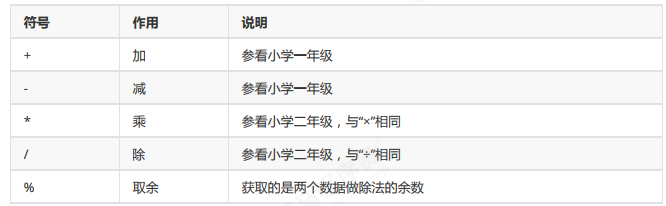```int a = 10;
int b = 3;
System.out.println(a / b); // 輸出結果3
System.out.println(a % b); // 輸出結果1```

char類型參與算術運算，使用的是計算機底層對應的十進制數值。需要我們記住三個字符對應的數值：

'a' -- 97 a-z是連續的，所以'b'對應的數值是98，'c'是99，依次遞加

'A' -- 65 A-Z是連續的，所以'B'對應的數值是66，'C'是67，依次遞加

'0' -- 48 0-9是連續的，所以'1'對應的數值是49，'2'是50，依次遞加

```// 可以通過使用字符與整數做算術運算，得出字符對應的數值是多少
char ch1 = 'a';
System.out.println(ch1 + 1); // 輸出98，97 + 1 = 98
char ch2 = 'A';
System.out.println(ch2 + 1); // 輸出66，65 + 1 = 66
char ch3 = '0';
System.out.println(ch3 + 1); // 輸出49，48 + 1 = 49```

byte類型，short類型和char類型將被提升到int類型，不管是否有其他類型參與運算。

```byte b1 = 10;
byte b2 = 20;
// byte b3 = b1 + b2; // 該行報錯，因為byte類型參與算術運算會自動提示為int，int賦值給byte可能損失

int i3 = b1 + b2; // 應該使用int接收
byte b3 = (byte) (b1 + b2); // 或者將結果強制轉換為byte類型
-------------------------------
int num1 = 10;
double num2 = 20.0;
double num3 = num1 + num2; // 使用double接收，因為num1會自動提升為double類型```

tips：正是由于上述原因，所以在程序開發中我們很少使用byte或者short類型定義整數。也很少會使用char類型定

`System.out.println("itheima"+ 666); // 輸出：itheima666`

```System.out.println(1 + 99 + "年黑馬"); // 輸出：199年黑馬
System.out.println(1 + 2 + "itheima" + 3 + 4); // 輸出：3itheima34
// 可以使用小括號改變運算的優先級
System.out.println(1 + 2 + "itheima" + (3 + 4)); // 輸出：3itheima7```

2.賦值運算符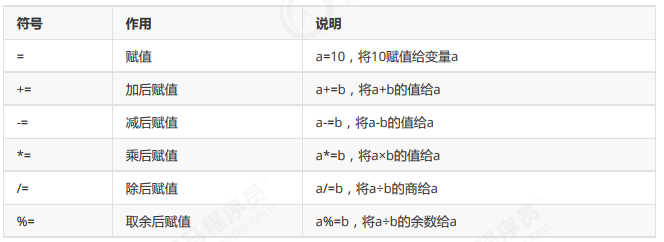```short s = 10;
s = s + 10; // 此行代碼報出，因為運算中s提升為int類型，運算結果int賦值給short可能損失精度
s += 10; // 此行代碼沒有問題，隱含了強制類型轉換，相當于 s = (short) (s + 10);```

3.自增自減運算符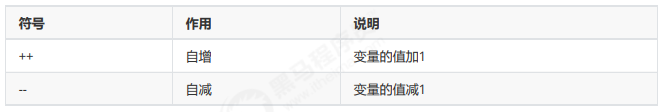++和-- 既可以放在變量的后邊，也可以放在變量的前邊。

```int i = 10;
i++; // 單獨使用
System.out.println("i:" + i); // i:11
int j = 10;
++j; // 單獨使用
System.out.println("j:" + j); // j:11
int x = 10;
int y = x++; // 賦值運算，++在后邊，所以是使用x原來的值賦值給y，x本身自增1
System.out.println("x:" + x + ", y:" + y); // x:11，y:10
int m = 10;
int n = ++m; // 賦值運算，++在前邊，所以是使用m自增后的值賦值給n，m本身自增1
System.out.println("m:" + m + ", m:" + m); // m:11，m:11```

4 .關系運算符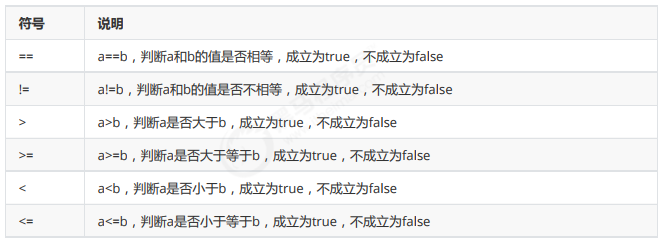```int a = 10;
int b = 20;
System.out.println(a == b); // false
System.out.println(a != b); // true
System.out.println(a > b); // false
System.out.println(a >= b); // false
System.out.println(a < b); // true
System.out.println(a <= b); // true
// 關系運算的結果肯定是boolean類型，所以也可以將運算結果賦值給boolean類型的變量
boolean flag = a > b;
System.out.println(flag); // 輸出false```

5.邏輯運算符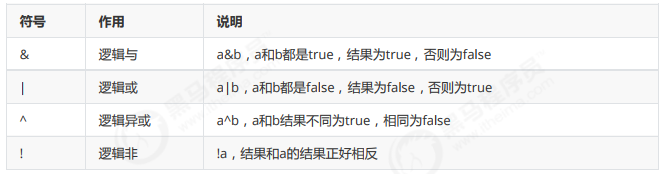```//定義變量
int i = 10;
int j = 20;
int k = 30;
//& “與”，并且的關系，只要表達式中有一個值為false，結果即為false
System.out.println((i > j) & (i > k)); //false & false,輸出false
System.out.println((i < j) & (i > k)); //true & false,輸出false
System.out.println((i > j) & (i < k)); //false & true,輸出false
System.out.println((i < j) & (i < k)); //true & true,輸出true
System.out.println("--------");
//| “或”，或者的關系，只要表達式中有一個值為true，結果即為true
System.out.println((i > j) | (i > k)); //false | false,輸出false
System.out.println((i < j) | (i > k)); //true | false,輸出true
System.out.println((i > j) | (i < k)); //false | true,輸出true
System.out.println((i < j) | (i < k)); //true | true,輸出true
System.out.println("--------");
//^ “異或”，相同為false，不同為true
System.out.println((i > j) ^ (i > k)); //false ^ false,輸出false
System.out.println((i < j) ^ (i > k)); //true ^ false,輸出true
System.out.println((i > j) ^ (i < k)); //false ^ true,輸出true
System.out.println((i < j) ^ (i < k)); //true ^ true,輸出false
System.out.println("--------");
//! “非”，取反
System.out.println((i > j)); //false
System.out.println(!(i > j)); //!false，,輸出true```

6.短路邏輯運算符```int x = 3;
int y = 4;
System.out.println((x++ > 4) & (y++ > 5)); // 兩個表達都會運算
System.out.println(x); // 4
System.out.println(y); // 5
System.out.println((x++ > 4) && (y++ > 5)); // 左邊已經可以確定結果為false，右邊不參與運算
System.out.println(x); // 4
System.out.println(y); // 4```

7.三元運算符

`關系表達式 ? 表達式1 : 表達式2;`

```int a = 10;
int b = 20;
int c = a > b ? a : b; // 判斷 a>b 是否為真，如果為真取a的值，如果為假，取b的值```

①需求：動物園里有兩只老虎，已知兩只老虎的體重分別為180kg、200kg，請用程序實現判斷兩只老虎的體重是 否相同。
```public class OperatorTest01 {
public static void main(String[] args) {
//1：定義兩個變量用于保存老虎的體重，單位為kg，這里僅僅體現數值即可。
int weight1 = 180;
int weight2 = 200;
//2：用三元運算符實現老虎體重的判斷，體重相同，返回true，否則，返回false。
boolean b = weight1 == weight2 ? true : false;
//3：輸出結果
System.out.println("b:" + b);
}
}```

②需求：一座寺廟里住著三個和尚，已知他們的身高分別為150cm、210cm、165cm，請用程序實現獲取這三個和尚的最高身高。

```public class OperatorTest02 {
public static void main(String[] args) {
//1：定義三個變量用于保存和尚的身高，單位為cm，這里僅僅體現數值即可。
int height1 = 150;
int height2 = 210;
int height3 = 165;
//2：用三元運算符獲取前兩個和尚的較高身高值，并用臨時身高變量保存起來。
int tempHeight = height1 > height2 ? height1 : height2;
//3：用三元運算符獲取臨時身高值和第三個和尚身高較高值，并用最大身高變量保存。
int maxHeight = tempHeight > height3 ? tempHeight : height3;
//4：輸出結果
System.out.println("maxHeight:" + maxHeight);
}
}```

CSS的三大特性介紹